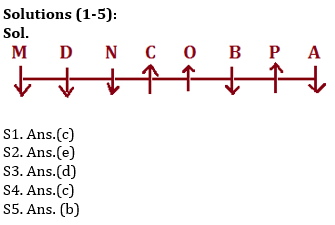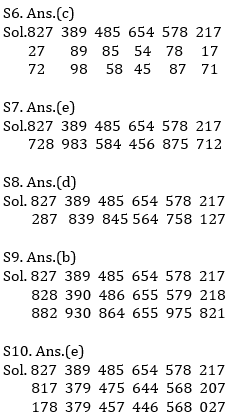Latest Banking jobs   »   NIACL AO 2021 Result Out, Mains Exam Date For 300 AO Recruitment   »   Reasoning Ability Quiz For IBPS Clerk/NIACL...

# Reasoning Ability Quiz For IBPS Clerk/NIACL AO Prelims 2021- 10th September

Directions (1-5): Read the following information carefully and answer the questions given below:
Eight persons A, B, C, D, M, N, O, and P are seating in a row, some are facing north and some are facing south but not necessarily in the same order. D sits 4th right of B. C sits left of N. M, D and N are facing in same direction. O doesn’t sit just near to N. A sits at one of the extreme ends and B sits 2nd to right of A. C and O are immediate neighbors of each other, none of them sits at any of the extreme end. M doesn’t sit just near to both B and N. Immediate neighbors of B face opposite direction of B. C and O face same direction but O doesn’t face south direction. N sits 3rd from one of the extreme ends.

Q1. Who among the following sits 2nd left of the one who sits immediate right of B?
(a) A
(b) M
(c) N
(d) C
(e) None of these

Q2. What is the position of A with respect to B?
(a) 4th to the left
(b) 3rd to the left
(c) Immediate left
(d) 4th to the right
(e) None of these

Q3. P is facing in which direction?
(a) North
(b) South
(c) Same as C faces
(d) Both a and c
(e) None of these

Q4. Who among the following sits immediate left of A?
(a) P
(b) B
(c) No one
(d) O
(e) None of these

Q5. How many persons sit to the left of C?
(a) One
(b) Three
(c) More than three
(d) Two
(e) None of these

Directions (6-10): These questions are based on the following six numbers.
827 389 485 654 578 217

Q6. If two digit numbers are formed by taking the 2nd and 3rd digits respectively of each of these numbers and then the two digits of each of these two digit numbers are interchanged, then which number from the given numbers will be the second highest number in the revised form?
(a) 389
(b) 485
(c) 578
(d) 827
(e) None of these

Q7. If these numbers are written with their digits in the reverse order, which number among them will be third highest?
(a) 485
(b) 578
(c) 217
(d) 389
(e) 827

Q8. If the first two digits of each number are interchanged, which among these numbers will be the 2nd lowest number?
(a) 485
(b) 645
(c) 217
(d) 827
(e) None of these

Q9. If one is added to each of the given numbers and then each digit is arranged in descending order within each number, then which of the following number is smallest?
(a) 485
(b) 654
(c) 578
(d) 217
(e) 827

Q10. If one is subtracted from middle digit in each given numbers and then all the digits in each number is arranged in ascending order then which of the following number is second smallest?
(a) 485
(b) 654
(c) 578
(d) 217
(e) 827

Directions (11-15): In these questions, relationship between different elements is show in the statements. The statements are followed by conclusions. Study the conclusions based on the given statements and select the appropriate answer:
(a) If only conclusion I follows.
(b) If only conclusion II follows.
(c) If either conclusion I or II follows
(d) If neither conclusion I nor II follows.
(e) If both conclusions I and II follow.

Q11. Statements: T<N≤P<O>R>Z=V≥I
Conclusion I: O>V      II: I<P

Q12. Statements: M<P≤C >I=G>Y=D>W
Conclusion I: M>Y      II: M≤Y

Q13. Statements: Z>S≥C>N =R>K<T=E>Y
Conclusion I: N=E       II: Z>K

Q14. Statements: A≤P=T≤U≥L>C=K>S≥V
Conclusion I: U≥A        II: V<U

Q15. Statements: T>W≤N=Z>D≥I>O≥K=J
Conclusion I: J<O          II: N≥I

SolutionsS11. Ans(a)
Sol.
I: O>V(True)         II: I<P(False)

S12. Ans(c)
Sol.
I: M>Y (False)        II: M≤Y(False)

S13. Ans(b)
Sol.
I: N=E(False)          II: Z>K(True)

S14. Ans(e)
Sol.
I: U≥A(True)          II: V<U(True)

S15. Ans(d)
Sol.
I: J<O(False)            II: N≥I(False)

Click Here to Register for Bank Exams 2021 Preparation Material#### Congratulations!Download Hindu Review of October 2021: Free PDF